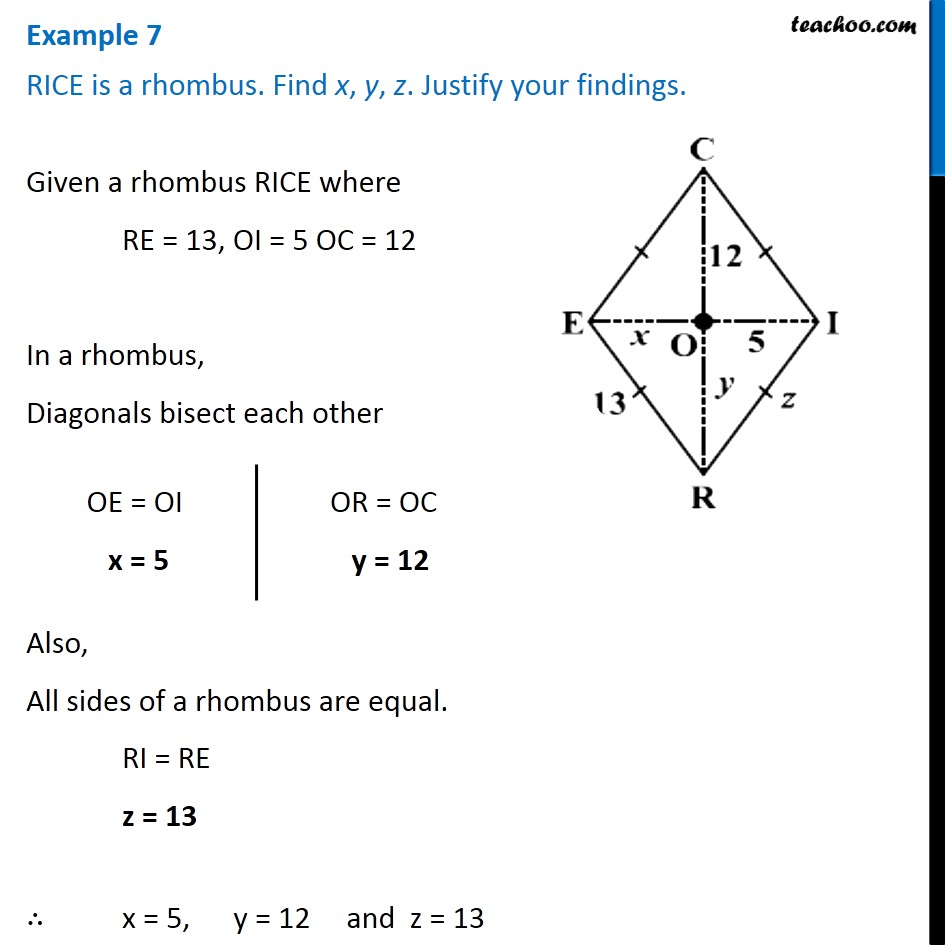Subscribe to our Youtube Channel - https://you.tube/teachoo

1. Chapter 3 Class 8 Understanding Quadrilaterals
2. Concept wise
3. Rhombus, Rectangle, Square

Transcript

Example 7 RICE is a rhombus. Find x, y, z. Justify your findings. Given a rhombus RICE where RE = 13, OI = 5 OC = 12 In a rhombus, Diagonals bisect each other OE = OI x = 5 OR = OC y = 12 Also, All sides of a rhombus are equal. RI = RE z = 13 ∴ x = 5, y = 12 and z = 13

Rhombus, Rectangle, Square